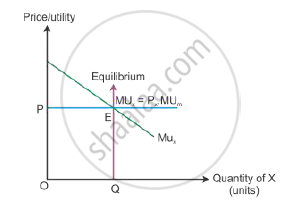# Given Price of a Goods, How Does a Consumer Decide as to How Much of the Good to Buy? - Economics

Given the price of a good, how does a consumer decide as to how much of the good to buy?

Given the price of a good, how will a consumer decide as to how much quantity to buy of that good? Explain.

Given the price of a good, how will a consumer decide as to how much quantity of that good to buy? Use utility analysis.

#### Solution

Given the price of the good, a consumer will decide the amount of goods to buy. So, the consumer compares the price of the good with its utility. A rational consumer will be at equilibrium only when the marginal utility is equal to the price paid for the good.
MUX = PX
The marginal utility is greater than the price paid for the good, i.e. MUX > PX implies that the consumer is not in equilibrium and buys more of a good. While the marginal utility is lesser than the price paid for the good, i.e. MUX < PX implies that the consumer is not in equilibrium and buys less of that good.In the diagram, OP is the price of the good given on the Y-axis and OQ is the utility given on the X-axis. The marginal utility curve MUX slopes downwards because the marginal utility
diminishes with every additional consumption of X. The consumer reaches equilibrium at Point E, where the marginal utility is equal to the price paid for the good

Concept: Consumer'S Equilibrium
Is there an error in this question or solution?